# larger heat exchanging

## large heat exchanging capacitance

For a large heat exchanging capacitance we need a large heat exchanging surface area of the separation wall. To this purpose the recuperator can be made large, the ducts small and each primary duct can be surrounded by secondary ducts and vice versa. By using ducts, halving the hydraulic diameter of the duct, has the same effect as doubling the diameter of the recuperator. The heat-exchanging surface is inversely proportional to the hydraulic diameter.

To increase the heat exchanging capacitance, the specific heat transfer through the wall can be increased. Therefore we need to increase the exchange of heat from the bulk of the flow to the wall. This can be achieved by mass flow, turbulent flow, from the bulk to the wall or through conduction, in laminar flow. In laminar flow we need a small distance from the bulk flow to the wall, so the temperature drop due to the heat conduction is low. The overall heat transfer coefficient is inversely proportional to the hydraulic diameter of the duct.
The heat exchanging capacitance is then inversely proportional to the square of the hydraulic diameter of the duct for laminar flow; so smaller ducts rapidly lead to high heat exchanging capacitance.

A very compact way of surrounding each primary duct by secondary ducts is using square or triangular ducts. Triangular ducts have the advantage over square ducts of showing a larger heat exchanging capacitance at the same pressure drop at a stable construction without overlap. The square shape leads to a mechanical instability and misalignment of the ducts. The plate shape (extreme elongated rectangular duct) leads also to a mechanical instable construction.

The triangular shape can be precisely produced, forming a mechanical stable product, with no loss due to misalignment.

If the overlap or misalignment is a translation, see fig. 1, the maximum reduction in heat exchanging surface is:

• square 50 %
• triangle none
•  plate none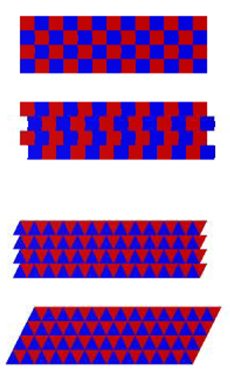Figure 1 reduction of heat exchanging surface due to overlap translation misalignment

The theoretical values can be obtained with a practical producible recuperator in the case of the triangle, but in the case of the square duct geometry an overlap of the ducts of minimal the material thickness is necessary and by misalignment at least an overlap of two is realized whereas the plate recuperator cannot be produced without spacers between the plates as otherwise the construction is totally unstable.

In figs. 2 to 10 the results at equal pressure drop for a minimum practical overlap for the square geometry and the minimum amount of spacers for the plate geometry are shown.
The heat exchanging capacitance is now 37 % higher for the triangle than for the square geometry, see fig. 2.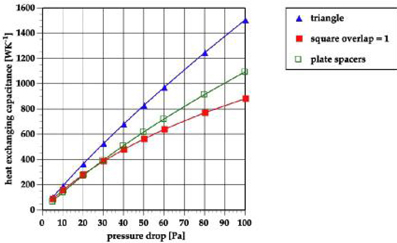Figure 2 comparison of the practical maximum heat exchanging capacitance of a 39 liter recuperator with triangular or square ducts and plates

The volume of the material for the foils is 48 % higher for the square duct geometry as for the triangular one and even 71 % higher for the plate configuration, see fig. 3.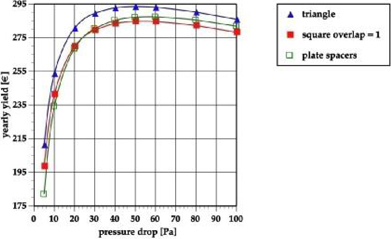Figure 3 comparison of the volume of the material used for the foils of a 39 liter recuperator with triangular or square ducts and a plate

The effectiveness of the triangle as compared to the practical maximum square is > 3 % higher, see fig. 4.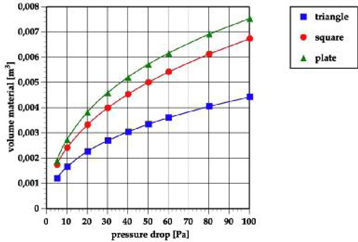Figure 4 comparison of the practical maximum effectiveness of a 39 liter recuperator with triangular or square ducts and plates

The yearly yield is about 3 % higher for the triangle than for the practical maximum square or plate, see fig. 5.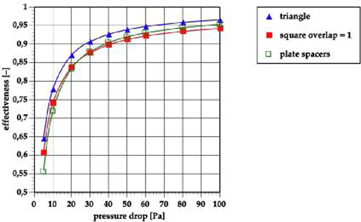Figure 5 comparison of the practical yearly yield of a 39 liter recuperator with triangular or square ducts

The net yearly yield of the triangle is 6 % higher than the practical square geometry, see fig. 6.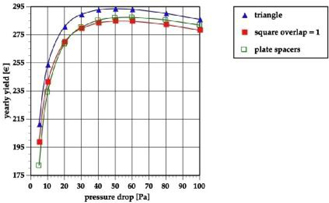Figure 6 comparison of the practical maximum net yearly yield of a 39 liter recuperator with triangular or square ducts and a plate

Though a difference of 4 % in effectiveness may seem small, it has a large effect on the comfort level as the temperature difference of the incoming fresh air with the resident air in the room becomes too high and is experienced as draught.

Only recuperators with triangular ducts deliver fresh air temperatures inside the comfort zone at maximum net yearly benefit!